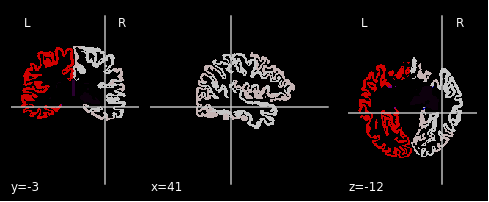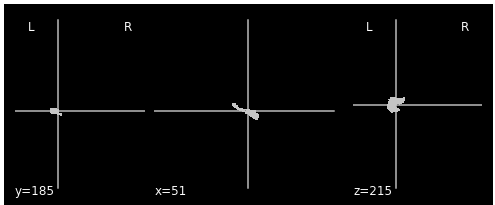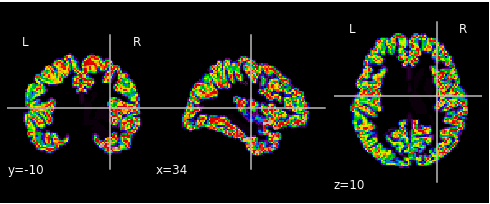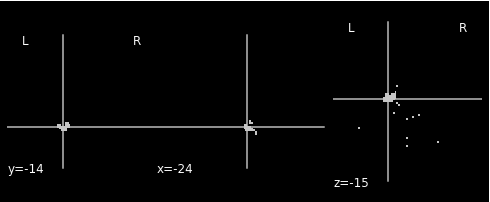# Transform ROI mask from Destrieux atlas to MNI using FLIRT

Hello all,

I’m trying to convert the ROI mask from Destrieux atlas to MNI space using fsl.FLIRT. The result looks off but I cannot seem to figure out why. Any insights would be extremely helpful, thank you all in advance!
I have each subject’s ROI mask on Destrieux atlas, which is the output of freesurfer with fmriprep. My plan is to first convert each subject’s Destrieux_seg ROI mask into MNI, then extract the bilateral hippocampus based on the labels in LUT.txt. My workflow runs, but the resulted ROI mask looks quite deprecated, as shown by those “flying” voxels. I believe the way I set up my fsl.FLIRT node is not idea, but I cannot figure out what exactly can be changed to make it better.

Here is my workflow, some unrelated nodes are not shown.

``````def _extract_roi(atlas, hemi, subj_id):

from nilearn import image
import numpy as np
import os

# load in the atlas in MNI space

# extract left or right HPC
if hemi == 'left':
HPC = 17
elif hemi == 'right':
HPC = 53
sub_hpc = np.where(atlas_img.get_data() == HPC, 1, 0)
sub_hpc_img = image.new_img_like(atlas_img, sub_hpc, affine=atlas_img.affine)

# resample to the resolution im using
reference_file = ref.nii.gz
roi_resample = image.resample_to_img(sub_hpc_img, reference_file, interpolation = 'nearest', clip = True)
roi_resample.to_filename(os.path.join(os.getcwd(),f'{subj_id}_{hemi}_hippo_resampled.nii.gz'))

return_path = os.path.join(os.getcwd(),f'{subj_id}_{hemi}_hippo_resampled.nii.gz')
return return_path

wf = pe.Workflow(name = 'create_hipp_roi')
wf.base_dir = os.path.join(output_dir,'working',subj_id)

# transfrom to MNI
flirt = pe.Node(
interface = fsl.FLIRT(),
name = 'to_mni')
flirt.inputs.output_type = 'NIFTI_GZ'
flirt.inputs.dof = 6
flirt.inputs.cost_func = 'mutualinfo'
flirt.inputs.reference = '/packages/fsl/5.0.10/install/data/standard/MNI152_T1_2mm.nii.gz'
flirt.inputs.out_file = f'{subj_id}_atlas.nii.gz'
flirt.interp = 'nearestneighbour'

# Get bilateral hippocampus and resample
extract_roi = pe.Node(
interface = niu.Function(
input_names = ['atlas','hemi', 'subj_id'],
output_names = ['roi'],
function = _extract_roi),
name = 'extract_roi')

(flirt, extract_roi, [('out_file', 'atlas')]),
(inputnode, extract_roi, [('hemi', 'hemi')]),
(inputnode, extract_roi, [('subj_id', 'subj_id')]),
(extract_roi, datasink, [('roi','result')])
])

# Run
wf.write_graph(graph2use='colored')
wf.run()
``````

This is the full Destrieux atlas for a given subject:This is the left hippocampus the Destrieux atlas:This is the atlas transformed into MNI space:This is the left hippocampus extracted from the transformed atlas above:As shown in the last figure, I think the MNI coordinates is generally ok for left hippocampus, but there are alots of extra floating voxels that dont make sense to me. I’m really confused here and would be grateful to any helps!